ID 772987
Date 12/04/2020
Public

## Basic Random Number Generators

Basic Random Number Generators (BRNG) are used to obtain random numbers of various statistical distributions. Non-uniform distribution generators depend on the quality of the underlying BRNGs. You should choose the BRNG depending on your application requirements, such as:

1. quality requirements

2. speed

3. memory use

4. efficient generation of random number subsequences

5. using random numbers as real ones

6. using random numbers as a bit stream

For example, a BRNG that cannot provide true randomness for lower bits is still applicable to applications using variates as real numbers.

VS provides a variety of BRNGs and permits you to register user-defined basic generators and use them in the same way as the BRNGs available with VS. You can also use random numbers generated externally, for example, from a physical source of random numbers [Jun99]. This makes VS a general-purpose library suitable for various tasks. See Abstract Basic Random Number Generators. Abstract Streams section for details.

Having several basic RNGs of different types available in VS also enables you to get more accurate verification results. Result verification is very important for computational experimentation. Typically, a researcher cannot verify the output since the solution is simply unknown. Any verification process involves testing of each structural element of the system. Being one of such structural elements, an RNG may produce inadequate results. To get more reliable results of the experiment, many authors recommend using several different BRNGs in a series of computational experiments.

VS provides the following basic pseudo-, quasi-, and non-deterministic random number generators:

BRNG Type Description
MCG31m1 pseudorandom A 31-bit multiplicative congruential generator.
R250 pseudorandom A generalized feedback shift register generator.
MRG32k3a pseudorandom A combined multiple recursive generator with two components of order 3.
MCG59 pseudorandom A 59-bit multiplicative congruential generator.
WH pseudorandom

A set of 273 Wichmann-Hill combined multiplicative congruential generators

(j = 1, 2, ... , 273 ).

MT19937 pseudorandom Mersenne Twister pseudorandom number generator.
MT2203 pseudorandom

A set of 6024 Mersenne-Twister pseudorandom number generators

(j = 1,...,6024).

SFMT19937 pseudorandom SIMD-oriented Fast Mersenne Twister pseudorandom number generator.
SOBOL (with Antonov-Saleev [Ant79] modification) quasi-random A 32-bit Gray code-based generator producing low-discrepancy sequences for dimensions 1≤s≤40
NIEDERREITER (with Antonov-Saleev [Ant79] modification) quasi-random A 32-bit Gray code-based generator producing low-discrepancy sequences for dimensions 1≤s≤318.
PHILOX4X32X10 pseudorandom A Philox4x32-10 counter-based pseudorandom number generator.
ARS5 pseudorandom A counter-based pseudorandom number generator, which uses instructions from the AES-NI set. Available in IA® architectures supporting this instruction set.
ABSTRACT pseudorandom or quasi-random, depending on the user-provided settings Abstract source of random numbers. See Abstract Basic Random Number Generators. Abstract Streams section for details.
NON-DETERMINISTIC Non-deterministic [BMT], available in the latest CPUs such as [AVX].

Each VS basic generator consists of the following subroutines:

1. Stream Initialization Subroutine. See section Random Streams and RNGs in Parallel Computation for details.

2. Integer Output Generation Subroutine. Every generated integral value (within certain bounds) may be considered a random bit vector. For details on randomness of individual bits or bit groups, see Basic Random Generator Properties and Testing Results.

3. Single Precision Floating-Point Random Number Vector Generation Subroutine. The subroutine generates a real arithmetic vector of uniform distribution over the interval [a, b).

4. Double Precision Floating-Point Random Number Vector Generation Subroutine. The subroutine generates a real arithmetic vector of uniform distribution over the interval [a, b).

The sections below discuss each basic generator in more detail and provide references for further reading.

### MCG31m1

32-bit linear congruential generators including MCG31m1 [L'Ecuyer99] are still used as the default RNGs in various systems because of the simplicity of implementation, speed of operation, and compatibility with earlier versions of the systems. However, their period lengths do not meet the requirements for modern BRNGs. Nevertheless, MCG31m1 has good statistical properties and may provide optimal results in generating random numbers of various distribution types for relatively small samplings.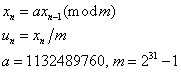### R250

R250 is a generalized feedback shift register generator. Feedback shift register generators have extensive theoretical footing and were first considered as RNGs for cryptographic and communications applications. Generator R250 proposed in [Kirk81] is fast and simple in implementation and is commonly used in the field of physics. However, the generator fails a number of tests, for example, a 2D self-avoiding random walk [Ziff98].### MRG32k3a

A combined generator MRG32k3a [L'Ecu99] meets the requirements for modern RNGs, such as good multidimensional uniformity or a fairly large period. Being optimized for various Intel® architectures, this generator rivals other VS basic RNGs in speed.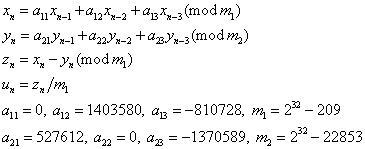### MCG59

A multiplicative congruential generator MCG59 is one of the two basic generators implemented in Numerical Algorithms Group (NAG) Numerical Libraries [NAG]. Since the module of this generator is not prime, its period length is 257 instead of 259, if the seed is an odd number. The drawback of such generators is that the lower bits of the output sequence are not random, therefore breaking numbers down into their bit patterns and using individual bits may be error-prone. For example, see [Knuth81], [L'Ecu94]. Besides, block-splitting of the sequence over the entire period into 2D similar blocks results in full coincidence of such blocks in d lower bits. For example, see [Knuth81], [L'Ecu94].### WH

Wichmann-Hill (WH) is a set of 273 different BRNGs. It is the second BRNG in NAG libraries. The constants ai,j are in the range from 112 to 127 and the constants mi,j are prime numbers in the range from 16718909 to 16776971, which are close to 224. These constants have been chosen so that they give good results with the spectral test, see [Knuth81] and [MacLaren89]. The period of each WH generator should be at least 292, but it is affected by common factors between (m1,j - 1), (m2,j - 1), (m3,j - 1), and (m4,j - 1). Still, each generator should have a period of at least 280. Further discussion of the properties of these generators is given in [MacLaren89], which shows that the generated pseudorandom sequences are essentially independent of one another according to the spectral test.NOTE:

The variables xn, yn, zn, wn in the above equations define a successive member of integer subsequence set by recursion. The variable un is the generator real output normalized to the interval (0, 1).

### MT19937

The Mersenne Twister pseudorandom number generator [Matsum98] is a modification of a twisted generalized feedback shift register generator proposed in [Matsum92], [Matsum94]. Properties of the algorithm (the period length equal to 219937).,,,,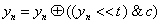,,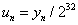,,,,,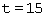,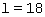,,,,,

with matrix A(32x32) having following format: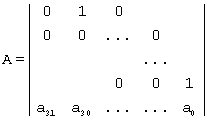where 32-bit vector a = a31...a0 has the value a = 0x9908B0DF.

### MT2203

The set of 6024 MT2203 pseudorandom number generators is an addition to MT19937 generator used in large-scale Monte Carlo simulations performed on distributed multi-processor systems. Parameters of the MT2203 generators are calculated using the methodology described in [Matsum2000] that provides mutual independence of the corresponding random number sequences. Every MT2203 generator has a period length equal to 22203.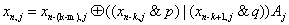,,,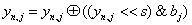,,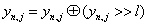,,,,,,,,,,

where matrix Aj(32x32) has the following format:,

with 32-bit vector aj = a31,j...a0,j.

### SFMT19937

The SIMD-oriented Fast Mersenne Twister pseudorandom number generator [Saito08] is analogous to the MT19937 generator and uses Single Instruction Multiple Data (SIMD) and multi-stage pipelining CPU features. SFMT19937 generator has a period of a multiple of 219937-1 and better equidistribution property than MT19937.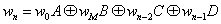where w0, wm, wn-2... are 128-bit integers, and are A, B, C, D sparse 128 x 128 binary matrices for which wA, wB, wC, wD operations are defined as follows:

1., left shift of 128-bit integer w by a followed by exclusive OR operation

2., right shift of each 32-bit integer in quadruple w by b followed by and-operation with quadruple of 32-bit masks mask, mask = (mask1mask2mask3mask4)

3.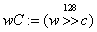, right shift of 128-bit integer w by c

4.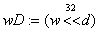, left shift of each 32-bit integer in quadruple w by d

5.,,k-th 32-bit integer in quadruple wn

6. Parameters of the generator take the following values: a = 8, b = 8, c = 8, d = 18, mask1=0xBFFFFFFG, mask2=0xBFFAFFFF, mask3=0xDDFECB7F, mask4=0xDFFFFFEF

### SOBOL

Bratley and Fox [Brat88] provide an implementation of the Sobol quasi-random number generator. VS implementation permits generating Sobol’s low-discrepancy sequences of length up to 232. This implementation also permits registering user-defined parameters (direction numbers or initial direction numbers and primitive polynomials) during the initialization, which allows obtaining quasi-random vectors of any dimension. If you do not supply user-defined parameters, the default parameter values [Brat88] are used for generation of quasi-random vectors. The default dimension of quasi-random vectors can vary from 1 to 40 inclusive.NOTE:

The value c is the right-most zero bit in n-1; xn is an s-dimensional vector of 32-bit values. The s-dimensional vectors (calculated during random stream initialization) vi, i = 1,32 are called direction numbers. Vector un is the generator output normalized to the unit hypercube (0,1)5.

### NIEDERREITER

According to the results of Bratley, Fox, and Niederreiter [Brat92] Niederreiter’s sequences have the best known theoretical asymptotic properties. VS implementation permits generating Niederreiter’s low-discrepancy sequences of length up to 232. This implementation also permits registering user-defined parameters (irreducible polynomials or direction numbers), which allows obtaining quasi-random vectors of any dimension. If you do not supply user-defined parameters, the default parameter values [Brat92] are used for generation of quasi-random vectors. The default dimension of quasi-random vectors can vary from 1 to 318 inclusive.### Philox4x32-10

This is a keyed family of counter-based BRNGs. The state consists of 128-bit integer counter c and two 32-bit keys k0 and k1. The generator output for each state consists of four 32-bit integer numbers obtained in the following way [Salmon2011]:

1. cn = cn-1 + 1
2. wn = f(cn), where f is a function that takes a 128-bit argument and returns a 128-bit number. The returned number is obtained as follows:
1. The argument c is interpreted as four 32-bit numbers, where, put k00 = k0 and k10 = k1.

2. The following recurrence is calculated: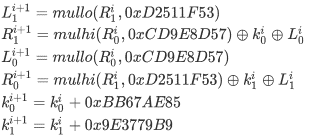Where mulhi(a,b) and mullo(a,b) are high and low 32-bit parts of the a*b product, respectively.

3. Put f(c) =, where N = 10

3. Integer output: r4n+k = wn(k), where wn(k) is the k-th 32-bit integer in quadruple wn, k = 0, 1, 2, 3

4. Real output: un = rn/232

### ARS5

ARS5 is a keyed family of counter-based BRNGs. The state consists of 128-bit integer counter c and a 128-bit key k. The BRNG is based on the AES encryption algorithm [FIPS-197]. The 32-bit output is obtained in the following way [Salmon2011]:

1. cn = cn-1 + 1
2. wn = f(cn), where f is a function that takes a 128-bit argument and returns a 128-bit number. The returned number is obtained as follows:
1. Put c0 = c xor k and k0 = k.
2. The following recurrence is calculated N times:
1. ci+1 = SubBytes(c)
2. ci+1 = ShiftRows(ci+1)
3. ci+1 = MixColumns(ci+1), this step is omitted if i + 1 = N
5. Lo(ki+1) = Lo(k) + 0x9E3779B97F4A7C15

Hi(ki+1) = Hi(k) + 0xBB67AE8584CAA73B

3. Put f(c) = cN, where N = 5

3. Integer output: r4n+k = wn(k), where wn(k) is the k-th 32-bit integer in quadruple wn, k = 0, 1, 2, 3

4. Real output: un = rn/232

Specification for the SubBytes, ShiftRows, MixColumns and AddRoundKey functions can be found in [FIPS-197].

### ABSTRACT

Abstract basic generators enable VS distribution generators to be used with the underlying uniform random numbers that are already generated. This feature might be useful in the following cases:

1. Random numbers of the uniform distribution are generated externally [Mars95]. For example, in a physical device [Jun99].

2. You want to study the system using the same uniform random sequence but under different distribution parameters [Mikh2000]. It is unnecessary to generate uniform random numbers as many times as many different parameters you want to investigate.

There might be other cases when abstract basic generators are useful. See Abstract Basic Random Number Generators. Abstract Streams section for further reading. Because of the specificity of abstract basic generators, you cannot use vslNewStream and vslNewStreamEx functions to create abstract streams. VS provides special vsliNewAbstractStream, vslsNewAbstractStream, and vsldNewAbstractStream functions to initialize integer, single precision, and double precision abstract streams, respectively.

### NON-DETERMINISTIC

This basic generator is an abstraction of the source of non-deterministic random numbers supported in hardware. A given hardware may support multiple non-deterministic sources. Each source supported by the library is associated with a unique identifier. By specifying the identifier during initialization of the basic generator, you can determine the non-deterministic random number generator to be used in the computations.

The current version of oneMKL provides the interface to RDRAND-based non-deterministic source only, defined by identifier VSL_BRNG_RDRAND [AVX], [IntelSWMan].

Some non-deterministic sources may require non-constant time to generate a random number. In this case, multiple requests to a non-deterministic source may be required before the next random number becomes available. In extreme cases, for example, when the non-deterministic source fails, the generator may require infinite time to get the next random number. To avoid such situations, the number of retries for requests to the non-deterministic source is limited to VSL_BRNG_NONDETERM_NRETRIES equal to 10. You can redefine the maximum number of retries during the initialization of the non-deterministic random number generator with the vslNewStreamEx function. For details on the non-deterministic source implementation for Intel® processors, see Chapter 7.3.18 in [IntelSWMan] and Chapter 4.2.2 in [BMT].

NOTE:

You can use non-deterministic random number generators only if the underlying hardware provides the respective support. For example, see Chapter 8 in [AVX] or Chapter 4 in [BMT] for instructions on how to determine whether an Intel® processor supports a non-deterministic random number generator.